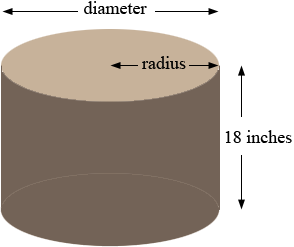SEARCH HOMEMath Central Quandaries & QueriesQuestion from Old: I have a round kettle I use to make soup for the hungry. I was curious on how many gallons it holds. The radius is 16 inches and the height is 18 inches.Hi,

Are you sure your kettle has a radius of 16 inches? That's a huge pot! I am going to assume that the diameter is 16 inches so the radius is 16/2 = 8 inches.The volume of a cylinder is given by π r2 h where r is the radius, h is the height and π is approximately 3.416. Your measurements are in inches so this will give the volume in cubic inches. You have r = 8 inches and h = 18 inches so that's

π × 82 × 18 = 3619.11 cubic inches.

There are 231 cubic inches in am American gallon so that is

3619.11/231 = 15.67 gallons.

I doubt that you fill the kettle to the top so I suggest that you replace the 18 inch height of the kettle by the height of the soup in the kettle and do the calculations again.

Keep up the good work,
PennyMath Central is supported by the University of Regina and The Pacific Institute for the Mathematical Sciences.